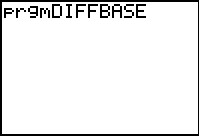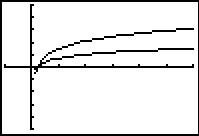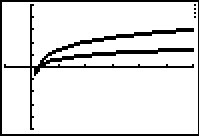••• ##### Device
• TI-83 Plus Family
• TI-84 Plus
• TI-84 Plus Silver Edition
•TI-84 Plus C Silver Edition
•TI-84 Plus CE
• ##### Report an Issue

Precalculus: Change Of Base
by Texas Instruments#### Overview

Discover the change of base rule for logarithms by examining the ratio of two logarithmic functions with different bases.

#### Key Steps

•In Problem 1, students use the program DIFFBASE to compare the graphs of two logarithmic functions with different bases.

•In Problem 2, students investigate the value of c, a constant factor, as the values of the bases of the logarithmic functions change.

•Students can then use the values of the bases and c stored in a table, to guess a formula for calculating c.